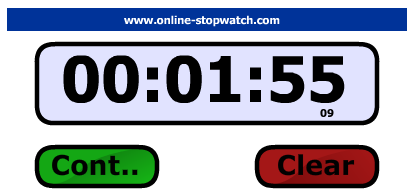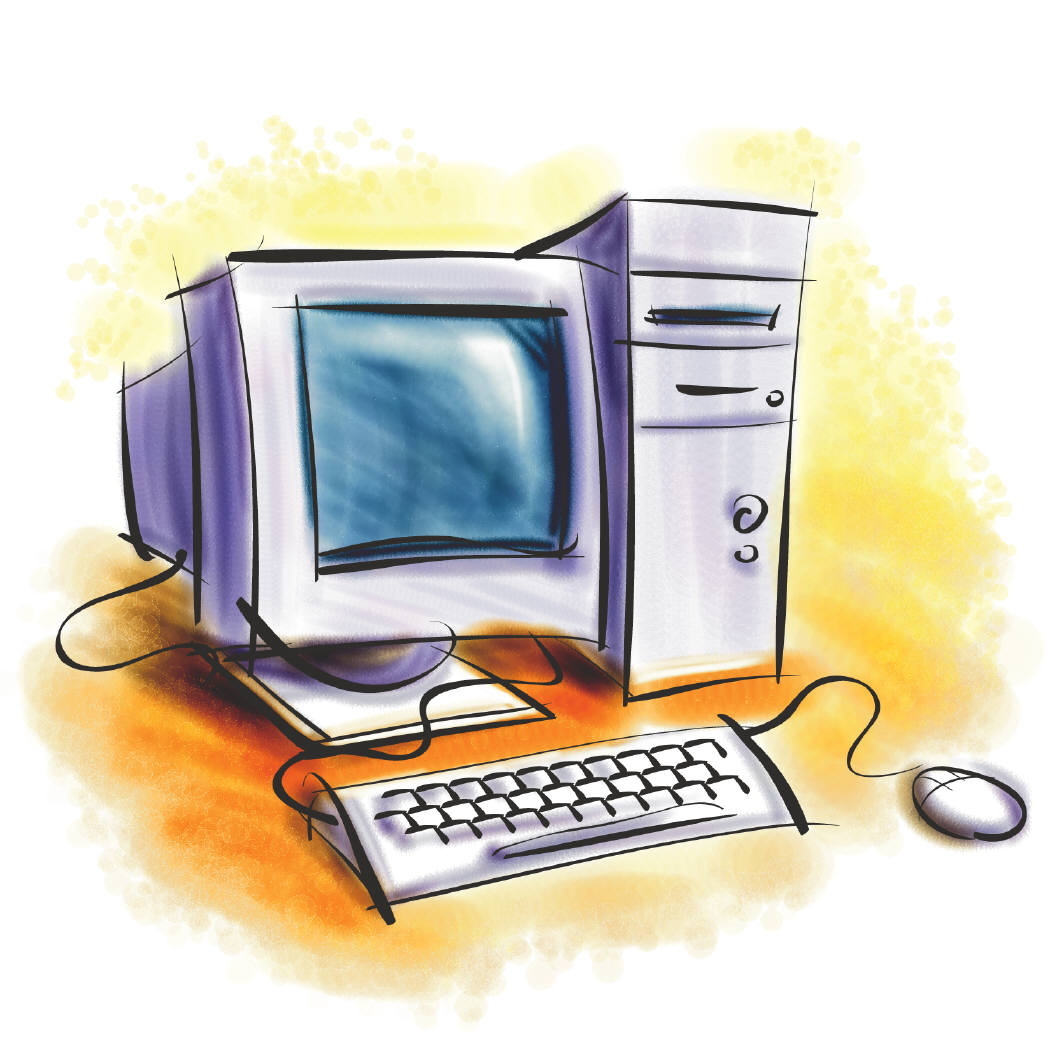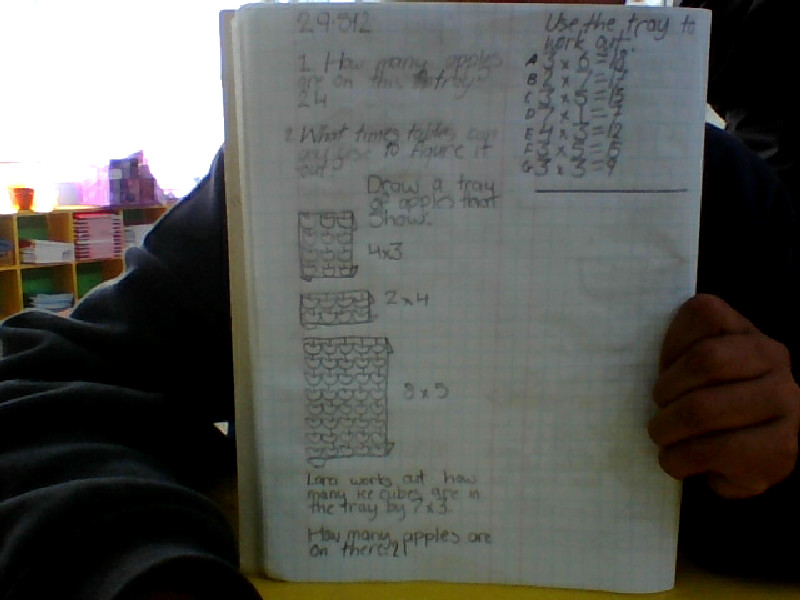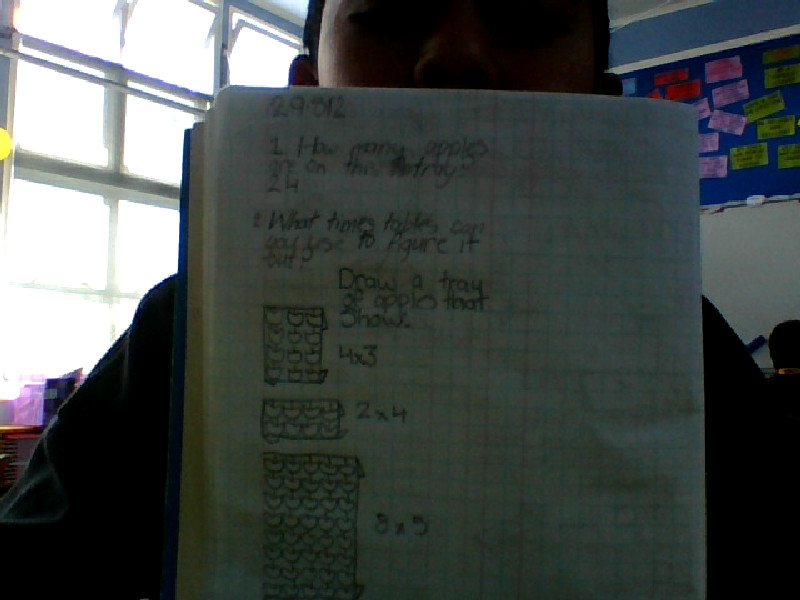Thursday, 4 December 2014

AccommodationMy Dream HolidayMonday, 1 December 2014

Christmas PoemThursday, 27 November 2014

¿Cuántos años tienes?WALT say how old we are in spanish

Tricky Time

WALT use quarter and half to tell the time.

Knowledge AttackWednesday, 26 November 2014

Coordinate ProfileWALT use coordinates to show position

Tuesday, 25 November 2014

Map of Tamaki PrimaryWALT use coordinates to show position
 se simple

Monday, 24 November 2014

Basic Facts: (T4W7)Thursday, 20 November 2014

Before, During, After ReadingWAL What to do before, during and after reading a text.

Wednesday, 19 November 2014

Basic Facts: (T4W6)

Basic Facts: (T4W6)
1. 9 + 1= 10
2. 40 - 10 = 30
3. 12 + 8 = 20
4. 1 + 9= 10
5. 66 - 6 = 60
6. 100 - 200 = 100
7. 100 + 30=130
8. 35 - 6=29
9. 14 + 14=28
10. 7 + 7=14
11. 4 + 5=9
12. 7 + 8=15
13. 2 x 9=18
14. 7 x 4=28
15. 5 x 2=10
16. 0 x 1=1
17. 11 x 9=99
18. 12 x 1=12
19. 3 x 4=12
20. 6 x 7=42
21. 4 x 2=8
22. 9 x 8=72
23. 6 x 6=36
24. 5 x 2=10
25. 9 x 9=81

Bear Hunt MapWALT put thing in order first to last.

Tuesday, 18 November 2014

Technology ChallengeWALT brainstorm blooms questions to research.
Today I was setting my bloom goals.

Monday, 17 November 2014

Positive Library behaviorWALT to identify positive behaviors in the library.

Friday, 14 November 2014

Multiplication Wheels!

WALT instantly recall our multiplication facts!

Knowledge AttackWALT do our basic fact faster.

Tuesday, 11 November 2014

Internet Scavenger HuntInternet Scavenger Hunt: Computers

Directions: What do you know about how computers work? Use the information
you find at the Web site provided below to answer the questions on this page.
Write your answers on the lines next to each question.

1. Which three parts of the computer receive input? Mouse,keyboard and modem

1. Which three parts of the computer give output? modem ,printer monitor screen

1. What does the computer hard disk do?It saves the computer files.

1. What does DOS stand for?Disk operating system

1. What kind of file has a name that ends with .gif?Graphics Interchange Format

1. In computer language, what does cd\ mean?compact data

Monday, 10 November 2014

Basic Facts: (T4W5)

Basic Facts: (T4W5)

1. 10 + 4= 14
2. 10 + 90= 100
3. 14 + 6= 20
4. 3 + 36= 39
5. 99 + 11= 110
6. 67 - 7= 60
7. 12 - 6 = 6
8. 7 - 2= 5
9. 100 - 55 = 45
10. 3 - 0= 3
11. 3 x 4=12
12. 6 x 7=42
13. 4 x 2=8
14. 9 x 8=72
15. 5 x 2=10
16. 1 x 10=10
17. 7 x 10=70
18. 3 x 8=24
19. 0 x 12=0
20. 3 x 3= 9
21. 2 x 10 = 20
22. 11 x 7 = 77
23. 9 x 8= 81
24. 4 x 3=12
25. 5 x 5 = 25
26. 2 + 5 + 3 + 5=15
27. 4 + 2 + 6 + 8=20
28. 0 + 9 + 7 + 3 + 1 + 10=30
29. 10 + 14 - 4 - 10=10

Tuesday, 4 November 2014

3D Detective

WALT identify properties of 3D shapes
Edge- The line where join/meet.
Vortex- A point where 2 or more straight lines meet.A corner.
2Dimension- Height Width.
Face- The flat surface of a space.
3Dimension-Width Height Depth
Success Criteria:

1.  I can find real life example of 3D shapes.
2. I know what 3D means.
3. I know what a face is .
4. I know what an edge is.
5. I know what a vertex is.
6. I can identify the face, edge and vertex of any 3D shape.

Friday, 24 October 2014

Knowledge AttackWALT get more quicker at our multiplication.

Thursday, 23 October 2014

Como estasWALT ask how are you.

Monday, 20 October 2014

Basic Facts: (T4W2)

Basic Facts: (T4W2)
1. 52 + 17 =69
2. 73 +15 =88
3. 61 + 26 =87
4. 99 - 32 =67
5. 57 - 25 =32
6. 16 - 4 =12
7. 35 + 32 =67
8. 23 + 53 =86
9. 72 + 15 =87
10. 67 - 41 =26
11. 41 + 27 =68
12. 25 + 52 =77
13. 39 - 14 =25
14. 40 + 33 =73
15. 13 - 5 =8
16. 55 ➗ 11 =5
17. 7 x 2 =14
18. 11 x 7 = 77
19. 3 x 9 =27
20. 12 x 3 =36
21. 63➗7=9
22. 5 x 7 =35
23. 72 ➗ 6 =12
24. 4 x 9 =36
25. 36 ➗ 6 =6
8 x 4 =32
81 ➗ 9 =9
27 ➗ 3 =9
9 x 3 =27
56 ➗ 7 =8

Thursday, 16 October 2014

What Technology Do I need?WALT understand that technology is driven by need.

My riddle:
I am walking on the concrete my feet are sore.What do I do?

Metui
Have you brushed your teeth lately, well if you haven't then get up and i’ll show you how to do it in the right way.Remember if you haven't brushed you your teeth ever then this is a good tutorial for you .

First you grab your toothbrush if you don't have one they sell it at supermarket and other places and make sure you’ve got toothpaste.Then after you got all that you are set to start.After you got all the stuff then you are ready.

To Start off you need to rinse your toothbrush this is because it either might be dried or to soften it.After that put some toothpaste on your toothbrush and then you start brushing your teeth.

Make sure you brush you teeth two times to make sure that it is shining and brush your tongue to give you fresh breath.After that when you finish you can put some water

Tuesday, 14 October 2014

Teacher Activity 1

47 + 6 =
WALT make tens + tidy number.
Today we were making tens and tidy number

Wednesday, 17 September 2014

Basic Facts: (T3W9)

Basic Facts: (T3W9)
1. 1/2 of 10=5
2. 1/5 of 25=5
3. 1/3 of 30=10
4. 1/4 of 12=3
5. 1/2 of 100=50
6. 1/3 of 15=5
7. 1/5 of 50=10
8. 10 + 50=6 0
9. 100 - 1=99
10. 59-13=46
11. 65-43=22
12. 67-11=56
13. 23+54=77
14. 79-52=27
15. 10 + 12=22
16. 77-23=54
17. 33+63=96
18. 86-33=53
19. 67-12=55
20. 20+45=65
21. 10x4=40
22. 10x10=100
23. 4x4=16
24. 5x3=15
25. 6x3=18
26. 10x6=60
27. 4x6=24
28. 3x7=21
29. 11x2=22
30. 6x5=30

Halves to 100

WALT identify our halves to 100 quickly!

Tuesday, 16 September 2014

What Comes Before?

\
WALT identify what number become before a number (to 1000).

Monday, 15 September 2014

Critical Thinking

Today our class were learning about using critical thinking
to show depth in our understanding of fiction books.
Here is our first try!

Monday, 8 September 2014

Map FactsWALT know our map facts.

Multiplication Wheels

WALT instantly recall our multiplication facts.

Basic Facts

Basic Facts: (T3W8)

1. 1 x 10= 10
2. 7 x 10= 70
3. 5 x 10= 50
4. 2 x 9= 18
5. 3 x 4= 12
6. 5 x 9= 45
7. 6 x 4= 24
8. 8 x 8= 64
9. 4 x 5 = 20
10. 7 x 4= 28
11. 20 + 5= 25
12. 22 + 5= 27
13. 90 + 20= 110
14. 100 + 200= 300
15. 50 + 50= 100
16. Double 20= 40
17. Double 40= 80
18. Half 70= 35
19. Half 100= 50
20. Double 500=1000

Friday, 5 September 2014

Multiplication Wheels

WALT instantly recall our multiplication facts.
I am trying to extend my learning.

WALT reflect on the book we read.

Blog Commenting

WALT blog the right way.
Here is a little information about blogging.
I posted three comment that I did.

Monday, 1 September 2014

Open and Closed QustionsWAL about open and closed questions

Basic FactsWALT complete the patterns on the drawing.

Friday, 29 August 2014

Blog Comment

WALT blog comment in the correct way.

Teacher Activity 2WALT solve timetables by doubling or halving.
This activity is related to the blog post be for thing.

Apple TraysThis is my apple trays, it is related to another activity and my teacher
has asked me to post it.

Ocean poemThursday, 28 August 2014

Basic Facts

Basic Facts: (T3W6)

1. 30 + 50=70
2. 70 + 30=100
3. 50 + 60=110
4. 100 + 20=120
5. 400 + 200=600
6. 2 x 10=20
7. 4 x 10=40
8. 2 x 8=16
9. 4 x 8=32
10. 5 x 5=25
11. 10 ÷ 2=5
12. 20 ÷ 2=10
13. 100 ÷ 2=50
14. 25 ÷ 5=5
15. 60 ÷ 3=10
16. 10 x 5=50
17. 2 x 4=8
18. 4 x 4=16
19. 36 ÷ 6=6
20. 5 x 2=10
21. 3 x 3=9
22. 20 x 3=60
23. 12 ÷ 2=6
24. 18 ÷ 3= 6
25. 500 + 500=100
26. 100 + 400=500
27. 300 + 600=900
28. 20 + 40=60
29. 1000 + 20=1020
30. 1000 + 100=1010